Question

Ohm’s Law is represented by the equation I=V/R. Explain how the current would change if the amount of resistance decreased and the voltage stayed the same. (HINT: try plugging numbers into the equation for R, but make sure V stays the same. Then describe what happens to I(current)

1.Latifah

Explanation:

The current will increase if the resistance is decreased and the voltage is kept constant. This is clearly seen from the mathematical relationship itself.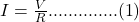This mathematical representation means that current I is inversely proportional to resistance R as long as V remains constant. By interpretation, if R increases I will decrease and vice-versa.

Let us assume a constant value of V = 5V and plug in different values of R, say R = 10, 5, 2 and 1 Ohms.

When R = 10 Ohm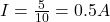When R = 5 Ohms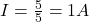When R = 2 Ohms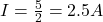When R = 1 Ohm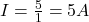It will be observed that as resistance decreases, current increases.